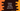# Python program to merge two lists and sort the merged list## Python 3 program to Merge two lists and sort the merged list:

Python list is one of the most commonly used datatypes. The list can hold different types of items. Each item can be accessed with its index. The index starts from 0, i.e. the index of the first element is 0, the index of the second element is 1 etc.

We can access any element using its index. List is mutable. We can delete and change any element of a list.

Square bracket ([]) is used for python lists. All items are placed inside a square bracket.

For accessing an item, a square bracket is used with the list name. The index of the element is placed in the square bracket like my_list. This will give us the element with index 0 in the list my_list.

Our problem is to merge two lists and sort the items of the final merged list. We will use only integer numbers in both lists to make the sorting easier.

Also, we will write the program to take the list values as inputs from the user. You can populate the lists with constant values at the starting of the program but a dynamic list will make the program more beautiful.

Before start writing the code, let’s take a look at the algorithm :

### The algorithm to merge two lists and sort the merged list:

1. Create two empty lists. We will populate these lists by taking the inputs from the user. To create an empty list, you can use an empty square bracket.

2. First, take the total number for the first list from the user. The total item count for both lists can be different. We are going to merge both lists, so the list size doesn’t matter.

3. Using a loop, read all the numbers for the first list and append them to the list. This loop will ask the user to enter the list item for each index one by one. The user will enter the number, our program will read it and append it to the list.

4. Similarly, take the total number for the second list and read all the numbers for the second list one by one from the user. We are using two loops here. The first loop will read and populate all the numbers for the first list, and the second list will do the same for the second list.

5. Append both lists and save it in a different variable. This is the final list.

6. Sort the final list. For sorting, we can implement our own sorting algorithm like selection sort, bubble sort, quick sort, etc. but python provides one inbuilt method for sorting the list items easily. We will use that one.

7. Print the final list to the user.

### Python3 Program :

``````first_list = []
second_list = []

#get total count for the first list
count_first_list = int(input("Enter total numbers of the first list : "))

#take inputs from the user for the first list
for i in range(1,count_first_list+1):
no = int(input("Enter : "))
first_list.append(no)

#get total count for the second list
count_second_list = int(input("Enter total numbers of the second list : "))

#take inputs from the user for the second list
for i in range(1,count_second_list+1):
no = int(input("Enter : "))
second_list.append(no)

#print first and second list
print("First list : ",first_list)
print("Second list : ",second_list)

#append both list
final_list = first_list + second_list
#sort the final list
final_list.sort()

#print the final sorted list
print("Final list : ",final_list)``````

The source code is available here.

### Notes :

1. We are using ‘for’ loop to read the numbers for the list. Two for loops are used here. The first one is for the first list, and the second one is for the second list.

2. To add an element to a list, ‘append()’ method is used.

3. To append both lists,‘+’ is used. It will merge both lists and returns the merged list.

4. To sort a list, ‘sort()’ is used. This method sorts all items in a list.